Introduction

Whenever a gas is in contact with a solid there will be an equilibrium established between the molecules in the gasphase and the corresponding adsorbed species (molecules or atoms) which are bound to the surface of the solid.

The Langmuir isotherm was developed by Irving Langmuir in 1916 to describe the dependence of the surface coverage of an adsorbed gas on the pressure of the gas above the surface at a fixed temperature. There are many other types of isotherm (Temkin, Freundlich ...) which differ in one or more of the assumptions made in deriving the expression for the surface coverage; in particular, or how they treat the surface coverage dependence of the enthalpy of adsorption. Whilst the Langmuir isotherme is one of the simples, it still provides a useful insight into the pressure dependence of the extent of surface adsorption.

The Langmuir isotherm - derivation from equilibrium considerations

The basic idea behind the Langmuir model is the coverage of the surface by a monomolecularic layer. The model approach also assumes that only one gas is being adsorbed. Thus, at constant temperature a part of the surface will be covered with the adsorbed molecule another part not.

Between the free gas and the adsorbed gas a dynamic equilibrium will exist. Per time unit there will be as much molecules adsorbing as there will be desorbing. The rate of adsorption will be proportional with the equilibrium pressure of the gas and the free surface.

In mathematical terms we derive the following model.

 Adsorption: Desorption: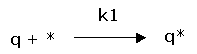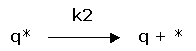(1) rate of adsorption: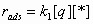(2) rate of desorption: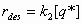(1) + (2)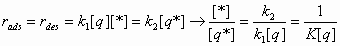With: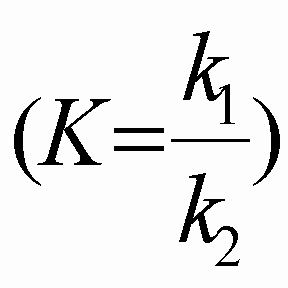Total number of adsorption sites: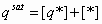Load factor or coverage or sorption capacity (3):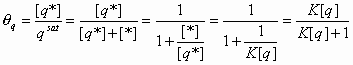Combining (3) and (4)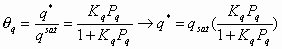(5) Applying the ideal gas law and assuming a Gas-Solid system (4):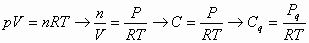(C is concentration)

Note that:
•   q --> KP at low pressures
•   q --> 1 at high pressures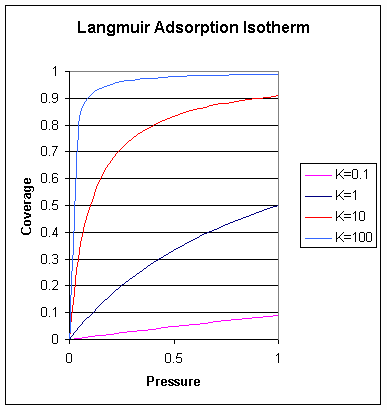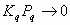Henry’s law i.e there is a linear relationship between the coverage and pressure.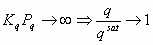The surface will be completely covered.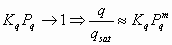(m<1), also called the Freundlich isotherm.

Criticism

The main problems with the model can be summarized as follows:

• One of the main assumptions used is that all adsorption sites are equally "active". This is not true. In a more refined model one would use a specific K for each specific site area.
• The influence, of already adsorbed molecules on lattice sites in the neigbourhood is neglected.
• Large molecules may occupy more than one adsorption site.
• The assumption that an adsorbed molecule is the same as a molecule in the gas phase is not necessarily valid. For example Hydrogen is adsorbed on Nickel as an atom, not as a molecule.
• Assuming that the adsorbed layer will be only one molecule thick is not valid. For example, if we calculate the amount if water which can be adsorbed in a zeolite, based on the surface area and monomolecular, the calculated capacity is much lower than the measured capacity.

The influence of the temperature on the adsorption equilibrium.

If we assume that the energy and the entropy of the lattice do not change significantly during adsorption than we can state that when a molecule is adsorbed the entropy will decrease, deltaS will be negative and therefore deltaH will be negative.

 Applying some thermodynamics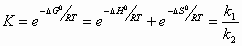Arrhenius: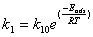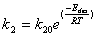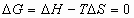at equilibrium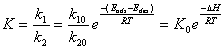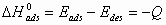At a given pressure the extent of adsorption is determined by the value of K: this in turn is dependent upon both the theperature (T) and the enthalpy (heat) of adsorption. The magnitude of adsorption ethalpy reflects the strengthn of binding of the adsorbate to the substrate.

K is increased by:

• a reduction in the system temperature
• an increase in the strength of adsorption

At higher temperature K reduces which means the surface becomes depopulated.

Chemisorption and Physisorption

With physical adsorption (physisorption) there is only an increase in surface concentration during adsorption. Only van der Waals forces play a role. The heat of adsorption is about 1-10 kcal/mole adsorbed gas.

With chemical adsorption (chemisorption) the heat of adsorption is anout 20-200 kcal/mole adsorbed gas. A chemical bond is formed between the molecule and the surface.

Not only the heat of adsorption but also the the activation energy of the adsorption process is higher with chemisorption than with physisorption. As a consequence physisorption is prelavent at lower temperatures and chemisorption at higher temperatures.

11/03/2002